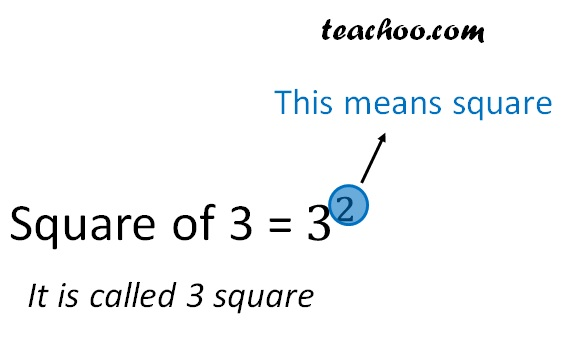Square numbers

Chapter 5 Class 8 Squares and Square Roots
Concept wise

Number = 3

Square of number = 3 2

= 3 × 3

= 9

Square numbers is a number which we get when a number is multiplied by itself 2 times.

Here, 9 is a square number

They are sometimes also called perfect squaresLet’s do some examples

 Number Square 1 1 × 1 = 1 2 2 × 2 = 4 3 3 × 3 = 9 4 4 × 4 = 16 5 5 × 5 = 25 6 6 × 6 = 36 7 7 × 7 = 49 8 8 × 8 = 64 9 9 × 9 = 81 10 10 × 10 = 100

Thus, 1, 4, 9, 16, 25, 36, 49, 64, 81, 100 are square numbers

### Finding Square of a Large Number

Find 23 2

23 2 = 23 ×  23

= (20 + 3) ×  (20 + 3)

= 20 ×  (20 + 3) + 3 ×  (20 + 3)

= 20 ×  20 + 20 ×  3 + 3 × 20 + 3 × 3

= 400 + 60 + 60 + 9

= 529

Find 39 2

39 2 = 39 ×  39

= (40 – 1) ×  (40 – 1)

= 40 × (40 – 1) – 1 ×  (40 – 1)

= 40 × 40 + 40 × (–1) – 1 × 40 – 1 × (–1)

= 1600 – 40 – 40 + 1

= 1601 – 40 – 40

= 1601 – 80

= 1521

### Is 1 a square number?

Square numbers is a number which we get when a number is multiplied by itself 2 times.

So, 1, 4, 9, 16, 25, 36, 49,  … are square numbers

∴ 1 is a square number

Learn in your speed, with individual attention - Teachoo Maths 1-on-1 Class